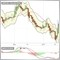How to retain local value of variable?231

I know, that in some cases non-buffered variables are lost. for example

static int MyVariable =0;

......

MyVariable =  (Condition ?   MyVariable+1  : MyVariable );

......

but in some cases i found (when something changed on chart or input or etc.. without refreshing the chart F5 ), the value of that variable is nulled.

I was advised to use buffers for such variables:

MyVariable[i] =  (Condition ?   MyVariable[i+1] +1  : MyVariable[i+1] );

however i have tens of such repeated variables in my indicators/scripts, and i cant set buffers for those variables... is any other solution? for example, what if i use array, will it be nulled too?

static int MyAr;

....

MyAr= (Condition ? MyAr+1 : MyAr);

....

any help?231

any help?2955

If your variable unexpectedly gets lost or nulled, then you have a bug in your code.19846

1. Definitely a bug in your code.
however i have tens of such repeated variables in my indicators/scripts, and i cant set buffers for those variables
Of course you can. But if you don't, you need their previous value(s) to do the current bar which is a problem with bar zero. Either buffers, only process bar zero once, or save and restore them when processing bar zero.
2. static int MyAr;
Why would define an array that only has one value?
3. MyAr= (Condition ? MyAr+1 : MyAr);

Why would you write such code? if(condition) ++MyArray; is simpler and 3 times faster.231

whroeder1:

thanks, i have a question, what means process bar zero only once? can you give me example, i dont understand what subject  (about zeros..) you are talking about....

In my indicator, if i left it in live chart, and clicked refresh, then many values of such "one-time" variable values were lost, and that's why i dont know what happened...  If i re-attached indi to chart, then values were correct.19846

1. "Only once?" I gave you the link. Either only use opening values
const int   lookback    = ...;
int      iBar  = Rates_total - MathMax(lookback, prev_calculated);
while(iBar > 0){  --iBar;
// calculate buffer[iBar]
}
#define  REDRAW_BAR_LAST false      // Don't redraw Bar zero.
return   rates_total - REDRAW_BAR_LAST - iBar;
or never update bar zero
const int   lookback    = ...;
int      iBar  = Rates_total - MathMax(lookback, prev_calculated);
while(iBar > 1){  --iBar;
// calculate buffer[iBar]
}
#define  REDRAW_BAR_LAST false      // Don't draw Bar zero.
return   rates_total - REDRAW_BAR_LAST - iBar;

2. Variables are not lost. If prev_calculated is zero, you must initialize them, you are not. Your code is broken, fix your broken code.